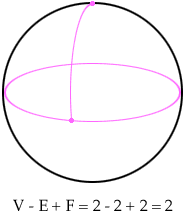Name: sandy Who is asking: Other Level: Elementary Question: How many faces are there on a sphere? What are the faces of a cone? What is the definition of a "face" of a 3D object? Hi Sandy, This reads like a question related to counts of vertices, edges, faces on various surfaces. (Euler's formula). So a sphere. Sometimes - to fit this setting, one imagines a sphere with one vertex on it. Then the entire sphere is one face, and there is one vertex so |V| - |E| + |F| = 2 as expected. You CAN of course, draw some additional curved 'edges' on the sphere, and have more faces (each additional edge either adds on more vertex or one more face - provided the edges and vertices are connected into a single piece).The idea here is that a 'face' should be somehow, in the sense of a stretchable rubber sheet (topologically) like a plane polygon. A sphere with NO vertices is just to different. Even if you really shrink the 'edges' of a rubber polygon, there will still be at least a point left of this 'edge' stuff. I think I have started to answer the third question as well. For the 'cone' - there are still some choices. I guess you probably mean the outside of a cone with a point at the bottom and a 'circle' thing on the top. If your "face' must be rather like a rubber polygon, and not a rubber 'ring', and an 'edge' must be like a rubber cord with two ends (perhaps with the ends held together), then you will need to 'see' (add?) a couple of things to the cone. First you need to add a point along the circle at the top (so the 'top' becomes an edge). THEN you need to somehow, connect the special point on the bottom, and the point on the top ring with another edge. Then you have: 2 vertices, 2 edges and 2 faces (the top and the sloped sides after being 'cut' by the edge from the point to the top). This IS a natural question. Sometimes mathematicians actually 'make up' (modify is more polite) their definitions to be sure that the ideas will work well for later work. This is what I am doing for a 'face' as the image of a rubber polygon, and an edge as the image of a rubber cord with two ends. People have studied such things for over 100 years, and have figured out that these kinds of objects give predictable results. Sometimes mathematicians actually disagree on these details. That is also part of how we work (but we don't usually talk about those things in YOUR books)! Sometimes two people look at the same thing and 'see' different ways to break it up into faces, vertices, edges. Look at the hood of a car. The designer may well have described it in the computer as a bunch of 'curved' triangles which are pasted together 'smoothly' along their edges. (That is the usual way in CAD/CAM.) But if they did it all well - YOU will not see where one triangle stopped and another started. You will see one single 'face' with a nice shine and a clear edge (maybe a curved polygon as the edge). BOTH are reasonable answers. The area of mathematics one is getting into here is called 'topology' (rubber sheet geometry is another good description). Very important in lots of ways. Walter Whiteley Go to Math Central